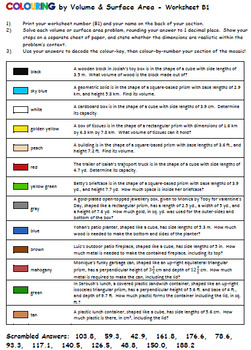DID YOU KNOW:
Seamlessly assign resources as digital activities

Learn how in 5 minutes with a tutorial resource. Try it Now

Learn More# Colouring by Volume and Surface Area of Prisms (16, 25, and 36 Sheet Mosaics)7th - 12th
Subjects
Standards
Resource Type
Formats Included
• Zip
Pages
166 pages

### Description

Collaborative motivation meets individual accountability in a highly engaging task involving surface area and volume application word problems. Each student's worksheet is different! Each worksheet represents a small section of the big picture!

This task makes a terrific unit summative assessment. It includes the volume and surface area of rectangular and triangular prisms.

CHECK OUT THE PRODUCT PREVIEW to see sample worksheets showing the range of problem types and letting you know exactly what these worksheets involve!

In a nutshell, students:

2. Colour the squares.

4. Combine with the class!

Students complete the problems to use their answers to colour-by-number their section of the mosaic.

INCLUDED:

-.pdf and .docx format files in zip folder

-Teaching Tips for smooth implementation of this task

-A complete answer key for quick student assessment of all worksheets

-An answer-range key, for example, "black [30, 43.1)" for at-a-glance evaluation.

-A picture of the completed mosaic with coordinates labelled to guide your assembly of the mosaic.

"Problem Order" (the order of problem types followed by all worksheets.)

1) volume of rectangular prism

2) volume of square-based prism

3) volume of cube

4) volume of rectangular prism

5) volume of square-based prism

6) volume of cube

7) volume of square-based prism

8) surface area of rectangular prism

9) surface area of cube

10) surface area of cube

11) surface area of equilateral triangular prism given perpendicular height

12) surface area of isosceles triangular prism given perpendicular height

13) surface area of rectangular prism

THE MATH INVOLVED:

-Interpret V and SA word problems arising from real life contexts

-Use volume formulas for prisms to solve problems

-Work with fractional and decimal edge lengths in the context of solving real-world problems

-Use both metric and imperial units of measurement for length, area, and volume, and consider the appropriateness of the (randomly chosen) unit for each problem.

-Consider the context of the problem (i.e. does the top area get included?)

Older students like colouring too! Their curiosity and desire to see the big picture come together will keep them working and helping each other!

If you like this product, be sure the check out the whole Collaborative Math Mosaic directory, sorted by topic!

All my "Colouring by..." math mosaics use the standard colours found in a Crayola 24 pack of coloured pencils. For best results, use the exact colour name match, and stick to one type of colouring medium. Maybe a class set of pencil crayons would be a fun departmental purchase? :)

~Calfordmath

Total Pages
166 pages
Included
Teaching Duration
2 days
Report this Resource to TpT
Reported resources will be reviewed by our team. Report this resource to let us know if this resource violates TpT’s content guidelines.

### Standards

to see state-specific standards (only available in the US).
Solve real-world and mathematical problems involving area, volume and surface area of two- and three-dimensional objects composed of triangles, quadrilaterals, polygons, cubes, and right prisms.
Represent three-dimensional figures using nets made up of rectangles and triangles, and use the nets to find the surface area of these figures. Apply these techniques in the context of solving real-world and mathematical problems.
Find the volume of a right rectangular prism with fractional edge lengths by packing it with unit cubes of the appropriate unit fraction edge lengths, and show that the volume is the same as would be found by multiplying the edge lengths of the prism. Apply the formulas 𝘝 = 𝘭 𝘸 𝘩 and 𝘝 = 𝘣 𝘩 to find volumes of right rectangular prisms with fractional edge lengths in the context of solving real-world and mathematical problems.